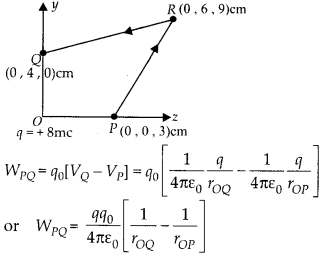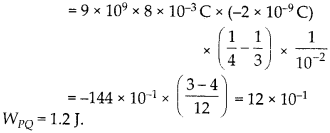Enlightened

# Acharge of 8 mC is located at theorigin. Calculate the work done in taking a small charge of – 2 × 10-9 C from a point P(0,0,3 cm) to a point Q (0,4 cm, 0), via a point R (0,6 cm, 9 cm).

• 0

Acharge of 8 mC is located at theorigin. Calculate the work done in taking a small charge of – 2 × 10-9 C from a point P(0,0,3 cm) to a point Q (0,4 cm, 0), via a point R (0,6 cm, 9 cm).

Share

1. Solution:

As electric field is conservative field, so work done in moving a charge in electric field is independent of path chosen to move the charge in electric field and depends only on the electric potential difference between the two end points. SoCheck the complete chapter with solutions.

NCERT Solutions for 12th Class Physics: Chapter 2-Electrostatic Potential and Capacitance

• 0